# MCQs on Power Systems

##### Page 10 of 67. Go to page 1 2 3 4 5 6 7 8 9 10 11 12 13 14 15 16 17 18 19 20 21 22 23 24 25 26 27 28 29 30 31 32 33 34 35 36 37 38 39 40 41 42 43 44 45 46 47 48 49 50 51 52 53 54 55 56 57 58 59 60 61 62 63 64 65 66 67
01․ Transmission line parameters of the short transmission line are
1,Z,Y,1
1,Z,0,1
1,0,Y,1
Z,0,Y,1

ABCD or transmission line parameters: Vs = AVr - BIr Is = CVr - DIr Where, Vs = Sending end voltage Vr = Receiving end voltage Is = Sending end current Ir = Receiving end current For short transmission line, line capacitance is neglected. Therefore, Ir = -Is, i.e., C = 0 and D = 1 Vs = Vr + Z Is i.e., A = 1 and B = Z

02․ Which of the following statements is/are true
Voltage regulation for short transmission line = (Vs - Vr) / Vr
Voltage regulation for medium transmission line = (Vs - Vr) / Vr
Voltage regulation for long transmission line = (Vs - Vr) / Vr
all of the above

Voltage regulation = (Vrnl - Vrfl)/Vrfl Vrnl = No load receiving end voltage Vrfl = full load receiving end voltage Vs = AVr + BIr At no load Ir = 0 Vs = A Vrnl Vrnl = Vs/A, Vrfl = Vr For short transmission line A = 1, therefore, Vrnl = Vs Voltage regulation for short transmission line = (Vs - Vr) / Vr

03․ The ABCD constants of a 3-phase transmission line are A = D = 0.8∠1°, B = 170∠85° Ω , C = 0.002∠90.4° â„§. The sending end voltage is 400 kV. The receiving end voltage under no load condition is
400 kV
500 kV
600 kV
350 kV

ABCD parameters Vs = AVr + BIr Is = CVr + DIr Where, Vs = Sending end voltage Vr = Receiving end voltage Is = Sending end current Ir = Receiving end current Under no load condition, Ir = 0 400 = 0.8∠1° * Vr Vr = 500∠-1° kV

400 MW
800 MW
1600 MW
200 MW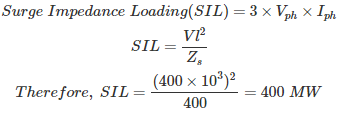Where, Vph = Phase voltage Iph = Phase current Vl = Line voltage Zs = surge impedance

05․ Surge impedance loading of a transmission line can be increased by
increasing its voltage level
addition of lumped inductance in parallel
addition of lumped capacitance in series
both 1 and 3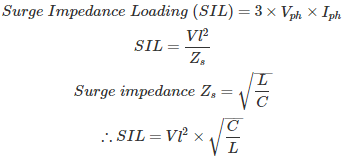Where, Vph = Phase voltage Iph = Phase current Vl = Line voltage Zs = surge impedance Therefore, SIL can be increased by increasing its voltage level. Zs can be decreased by the addition of lumped capacitance in series which reduces the effective reactance of the line resulting into higher SIL.

06․ For transmission line which one of the following relation is true?

For transmission line, Symmetry network A = D Reciprocity network AD - BC = 1

07․ A 3-phase transmission line has its conductors at the corners of an equilateral triangle with side 3m. The diameter of each conductor is 1.63 cm. The inductance of the line per phase per km is
1.093 mH
1.043 mH
1.182 mH
1.232 mH

Inductance of a transmission line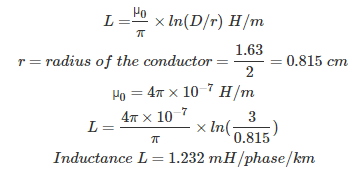Where, d = distance between the conductors = 3m r' = 0.7788 r

08․ For some given transmission line the expression for voltage regulation is given by (Vs - Vr)/Vr *100%. Hence,
this must be a short line
this may either be a medium line or short line
this expression is true for any line
none of the above

Voltage regulation = (Vrnl - Vrfl)/Vrfl Vrnl = No load receiving end voltage Vrfl = full load receiving end voltage Vs = AVr + BIr At no load Ir = 0, Vs = A Vrnl Vrnl = Vs/A, Vrfl = Vr For short transmission line A = 1, therefore, Vrnl = Vs Voltage regulation for short transmission line = (Vs - Vr)/Vr

09․ The capacitance of an overhead transmission line increases with
increase in mutual geometrical mean distance
increase in height of conductors above ground
both 1 and 2
neither 1 nor 2

Capacitance of a transmission line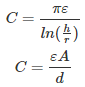Where, h = height of conductors above ground r = radius of the conductors ε = permittivity Therefore, as the height of the conductor increases from the ground, the capacitance will also decrease. Where A = area d = geometrical mean distance Therefore, as an increase in mutual geometrical mean distance, the capacitance will decrease. Therefore, both statements are wrong statements.

10․ The charging reactance of 50 km transmission line is 1500 Ω. What is the charging reactance for 100 km length of line?
1500 Ω
3000 Ω
750 Ω
1000 Ω

Charging reactance Xc = 1/ωC ∝ 1/ length as C ∝ length Charging reactance is inversely proportional to length of the trasnmission line. Therefore, new charging reactance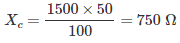<<<89101112>>>